# Bearings

GCSELevel 4-5AQACambridge iGCSEEdexcelEdexcel iGCSEOCRWJEC

## Bearings

Bearings are a way of expressing the angle between two objects. There is a specific set of rules about how bearings should be calculated and expressed.

1. Always measure bearings from the North line.

2. Always express your answers as three-figure bearings (so $60\degree$ would be $060\degree$).

3. Always draw and measure bearings clockwise.

Understanding angles on parallel lines is required for this topic.

Level 4-5GCSEAQAEdexcelOCRWJECCambridge iGCSEEdexcel iGCSE

## Skill 1: Bearings – 3 Rules

There is a specific set of rules about how bearings should be calculated and expressed.

1. Always measure bearings from the North line.

2. Always express your answers as three-figure bearings (so $60\degree$ would be $060\degree$).

3. Always draw and measure bearings clockwise.

Level 4-5GCSEAQAEdexcelOCRWJECCambridge iGCSEEdexcel iGCSE

## Example 1: Measure a Bearing

Find the bearing of $\textcolor{blue}{B}$ from $\textcolor{red}{A}$.

[1 mark]

Note: the terminology “$B$ from $A$” is always used, as opposed to “$A$ to $B$”.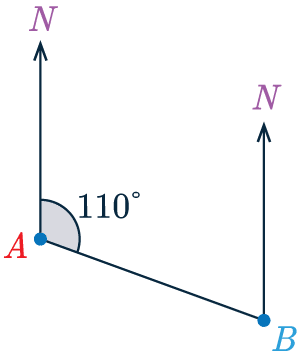So, we have our two points and a North line coming off both.

The bearing of $\textcolor{blue}{B}$ from $\textcolor{red}{A}$ is measured from the North line going clockwise until we hit the straight line.

Then using a protractor, we measure the angle to be $110\degree$ which is the bearing of $\textcolor{blue}{B}$ from $\textcolor{red}{A}$.

Level 4-5GCSEAQAEdexcelOCRWJECCambridge iGCSEEdexcel iGCSE

## Example 2: Drawing Bearings

Two boats $\textcolor{red}{A}$ and $\textcolor{blue}{B}$ are $5$km apart, and the bearing of $\textcolor{blue}{B}$ from $\textcolor{red}{A}$ is $256\degree$.

Using the scale $1\text{ cm}:1\text{ km}$, construct a diagram showing the relative positions of points $\textcolor{red}{A}$ and $\textcolor{blue}{B}$.

[2 marks]

First, we draw point $\textcolor{red}{A}$ with a North line and measure an angle of $104\degree$ going anticlockwise from it (This is because $360 - 254 = 104\degree$. You can’t measure $256\degree$ using a protractor any other way).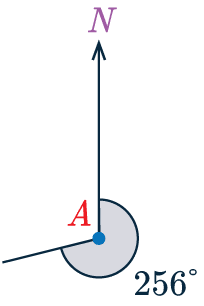Then, as $\textcolor{red}{A}$ and $\textcolor{blue}{B}$ are $5$ km apart, we will need to make the line from $\textcolor{red}{A}$ to $\textcolor{blue}{B}$ (going along the bearing we’ve determined) $5$ cm long.

The result of this is below, not drawn accurately.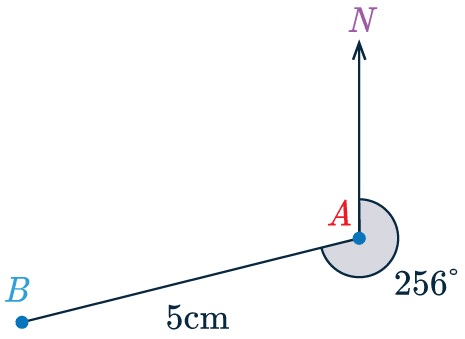Level 4-5GCSEAQAEdexcelOCRWJECCambridge iGCSEEdexcel iGCSE

## Example 3: Finding Bearings

The diagram below shows the bearing of $\textcolor{blue}{B}$ from $\textcolor{red}{A}$.

Find the bearing of $\textcolor{red}{A}$ from $\textcolor{blue}{B}$.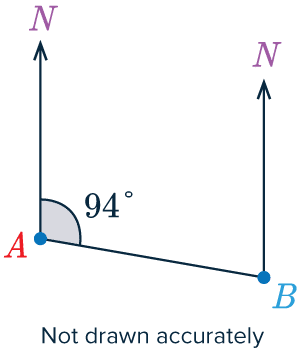[2 marks]

Now, we can’t measure the angle because the diagram is not drawn accurately.

We will use the fact that both North lines are parallel and extend the line $\textcolor{red}{A}\textcolor{blue}{B}$ past point $\textcolor{blue}{B}$, the angle formed by the North line at $\textcolor{blue}{B}$ and the extension to line $\textcolor{red}{A}\textcolor{blue}{B}$ and the bearing of $\textcolor{blue}{B}$ from $\textcolor{red}{A}$ are corresponding angles (also known as an “F angle”).

So, from our knowledge of parallel lines, we know that they must be equal.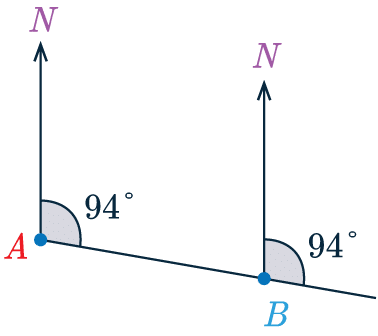Finally, we are measuring the line of $\textcolor{red}{A}$ from $\textcolor{blue}{B}$ so we need to go clockwise from the north line at $\textcolor{blue}{B}$ to the line $\textcolor{red}{A}\textcolor{blue}{B}$.

We have $94\degree$ but need the remaining portion of the angle.

Fortunately, the remaining portion of the angle is just a straight line, so the bearing of $\textcolor{red}{A}$ from $\textcolor{blue}{B}$ is

$94 + 180 = 274\degree$

Level 4-5GCSEAQAEdexcelOCRWJECCambridge iGCSEEdexcel iGCSE

## Bearings Example Questions

Let the lighthouse be L and the boat be B. As we’re finding the bearing of L from B, we shall measure an angle of $051\degree$ clockwise at B.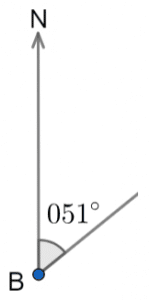Then, as B and L are 70 miles apart, we will need to make the line from B to L 7cm long. The final diagram should look like,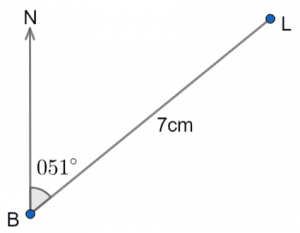Gold Standard Education

We can find the other angle around the point B by subtracting 295 from 360,

$360\degree - 295\degree = 65 \degree$

Then, because the two North lines are parallel, we can say that the bearing of B from A and the $65\degree$ angle we just found are co-interior. These two angles (marked with red below) must add to 180.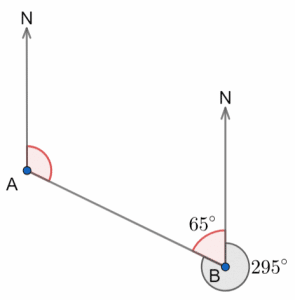So, we get:

$\text{Bearing of B from A } = 180\degree - 65\degree = 115\degree$Gold Standard Education

Drawing straight lines along each of the bearings, we can find C at  the point of intersection of both lines.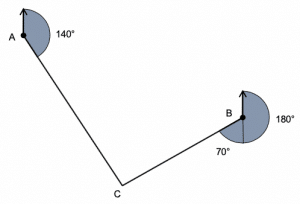Gold Standard Education

By use of a protractor or otherwise  we find the angle of 60 degrees. This written as a bearing is,

$060 \degree$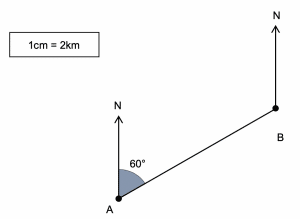Gold Standard Education

The two North lines are parallel, so we can say that the bearing of B from A and the co-interior angle at B must add to 180 degrees. Thus the co-interior angle is

$180 \degree-60\degree = 120 \degree$

As angles around a point sum to 360 degrees we can find the bearing of A from B as,

$360 \degree-120 \degree=240 \degree$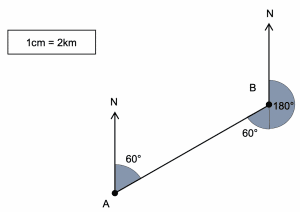Gold Standard Education

## Bearings Worksheet and Example Questions

### (NEW) Bearings Exam Style Questions - MME

Level 4-5GCSENewOfficial MME

## Bearings Drill Questions

### Bearings - Drill Questions

Level 4-5GCSEGold Standard Education

£19.99 /month. Cancel anytime

Level 4-5GCSEKS3